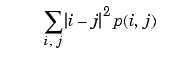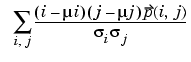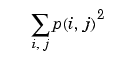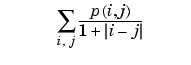Image Processing Toolbox User's Guidegraycoprops

Properties of a gray-level co-occurrence matrix

Syntax

• ```stats = graycoprops(glcm, properties)
```

Description

```stats = graycoprops(glcm, properties) ``` calculates the statistics specified in `properties` from the gray-level co-occurence matrix `glcm`. `glcm` is an m-by-n-by-p array of valid gray-level co-occurrence matrices. If `glcm` is an array of GLCMs, `stats` is an array of statistics for each `glcm`.

`graycoprops` normalizes the gray-level co-occurrence matrix (GLCM) so that the sum of its elements is equal to `1`. Each element (r,c) in the normalized GLCM is the joint probability occurrence of pixel pairs with a defined spatial relationship having gray level values r and c in the image. `graycoprops` uses the normalized GLCM to calculate `properties`.

`properties` can be a comma-separated list of strings, a cell array containing strings, the string `'all'`, or a space separated string. The property names can be abbreviated and are not case sensitive.

 Property Description Formula `'Contrast'` Returns a measure of the intensity contrast between a pixel and its neighbor over the whole image. ```Range = [0 (size(GLCM,1)-1)^2] ``` Contrast is 0 for a constant image. `````` `'Correlation'` Returns a measure of how correlated a pixel is to its neighbor over the whole image. `Range = [-1 1]` Correlation is 1 or -1 for a perfectly positively or negatively correlated image. Correlation is `NaN` for a constant image. `````` `'Energy'` Returns the sum of squared elements in the GLCM.```Range = [0 1] ``` Energy is `1` for a constant image. `````` `'Homogeneity'` Returns a value that measures the closeness of the distribution of elements in the GLCM to the GLCM diagonal. ```Range = [0 1] ``` Homogeneity is 1 for a diagonal GLCM. ``````

`stats` is a structure with fields that are specified by `properties`. Each field contains a 1 x p array, where p is the number of gray-level co-occurrence matrices in GLCM. For example, if GLCM is an 8 x 8 x 3 array and properties is `'Energy'`, then `stats` is a structure containing the field Energy, which contains a 1 x 3 array.

Notes

Energy is also known as uniformity, uniformity of energy, and angular second moment.

Contrast is also known as variance and inertia.

Class Support

`glcm` can be logical or numeric, and it must contain real, non-negative, finite, integers. `stats` is a structure.

Example

• ```GLCM = [0 1 2 3;1 1 2 3;1 0 2 0;0 0 0 3];
stats = graycoprops(GLCM)

`graycomatrix`graycomatrix grayslice